MATLAB模型预测控制（MPC,Model Predictive Control）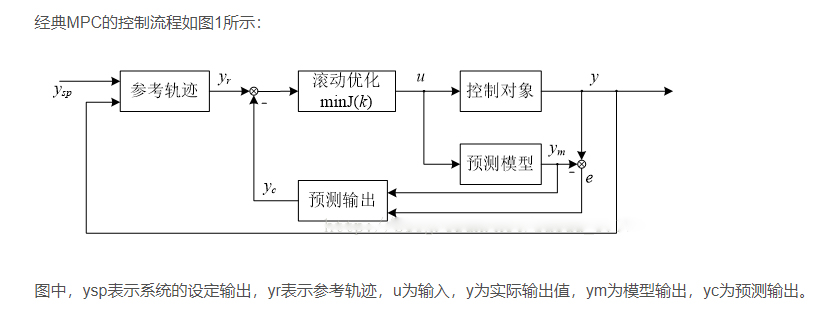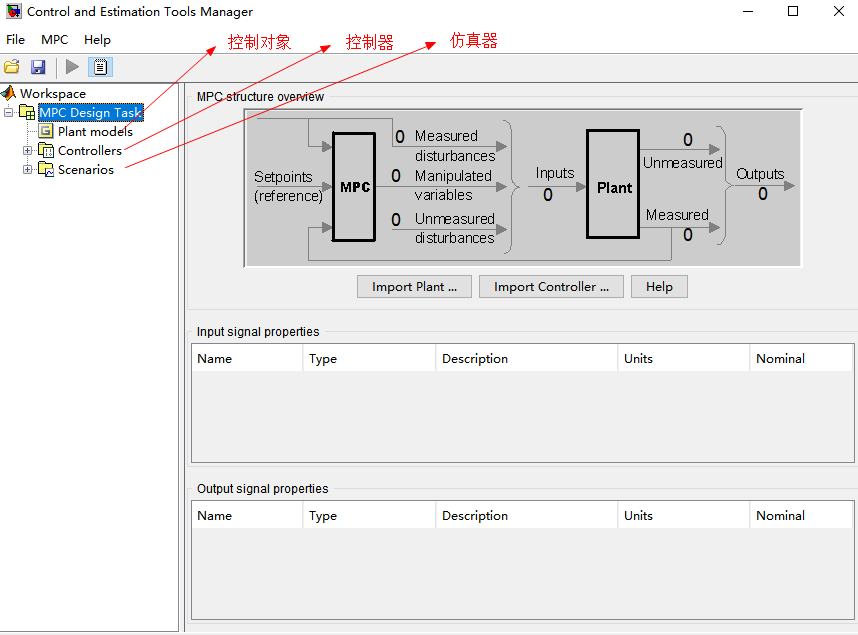Mo(measured output):当前可测量的输出信号
Ref(Reference signa):参考信号
Md(optional measured disturbance signa):可选的测量干扰信号
Mv（optimal manipulated variables ）：最优操纵变量
MPC一般通过求解一个二次规划(这里我们使用ord45)来计算最优操纵变量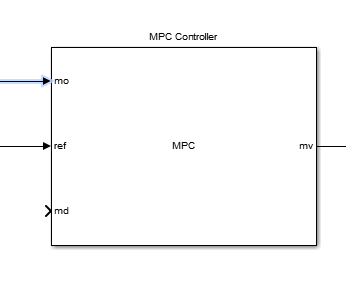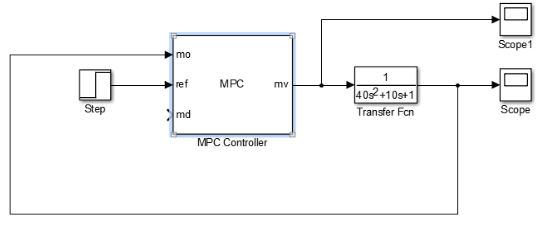多个MPC调度控制器解决方案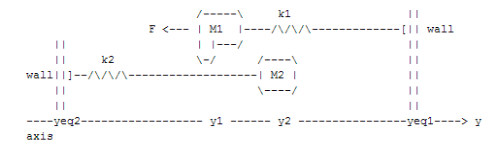%模型参数%
clear all clc M1
=1; % M1的质量 M2=5; % M2的质量 k1=1; % 弹簧k1常数k k2=0.1; % 弹簧k2常数k b1=0.3; % 与空气的摩擦系数，与速度成正比 b2=0.8; % 摩擦系数 yeq1=10; % 刚性壁1的位置 yeq2=-10; % 刚性壁2的位置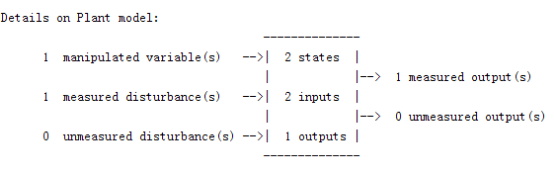states：M1的位置和速度
MV：推力F
MD：物体１由弹簧的需求的力Ｆ和实际给出的Ｆ之间的常量误差,用来弥补弹簧弹力，使M在初始位置0上时，弹簧对其作用力为零。
MO：M1的位置

%% 状态空间模型
% 当M1和M2不接触时候的状态模型.
A1=[0 1;-k1/M1 -b1/M1];
B1=[0 0;-1/M1 k1*yeq1/M1];
C1=[1 0];
D1=[0 0];
sys1=ss(A1,B1,C1,D1);                 %建立状态空间表达式
sys1=setmpcsignals(sys1,'MD',2);    %第二个输入是可测量扰动
%%Setmpcsignals：设置MPC受控对象的的I/0信号模型。这个系统必须是线性时不变系统
%’MD’：可测量的输入扰动，，第二个输入是可测量的输入扰动。如果没有指定的输入变量是MV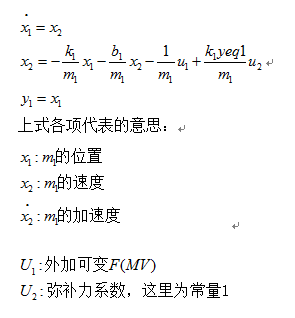% 当M1和M2接触时候的状态模型.
A2=[0 1;-(k1+k2)/(M1+M2) -(b1+b2)/(M1+M2)];
B2=[0 0;-1/(M1+M2) (k1*yeq1+k2*yeq2)/(M1+M2)];
C2=[1 0];
D2=[0 0];
sys2=ss(A2,B2,C2,D2);
sys2=setmpcsignals(sys2,'MD',2); %第二个输入是可测量扰动
%%Setmpcsignals：设置MPC受控对象的的I/0信号模型。这个系统必须是线性时不变系统
%’MD’：可测量的输入扰动，，第二个输入是可测量的输入扰动。如果没有指定的输入变量是MV
%%Multi-MPC控制设置
%%
MPC仿真参数设置 Ts=0.2; % 采样时间 p=20; % 预测时域长度 m=1; % 控制时域长度 %% 建立MPC模型 MPC1=mpc(sys1,Ts,p,m); % 定义M1和M2分离的MPC模型 % 设置限制，F的大小不能为0，F斜率绝对值最大1000 MPC1.MV=struct('Min',0,'Max',Inf,'RateMin',-1e3,'RateMax',1e3); MPC2=mpc(sys2,Ts,p,m); %定义M1和M2连接的MPC2模型 MPC2.MV=MPC1.MV; %系统1和系统2的控制限制一样
%% 仿真参数设置
Tstop=100;             % 仿真时间
y1initial=0;           % 物体1的初始位置
y2initial=10;          % 物体2的初始位置
open_system('wc1');   % 调用wc1.MDL
disp('Start simulation by switching control between MPC1 and MPC2 ...');
set_param('wc1/signals','Open','On');%设置系统和模型的参数值
sim('wc1',Tstop);%开始动态系统仿真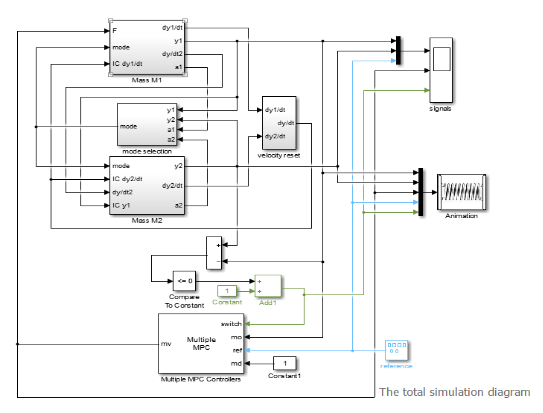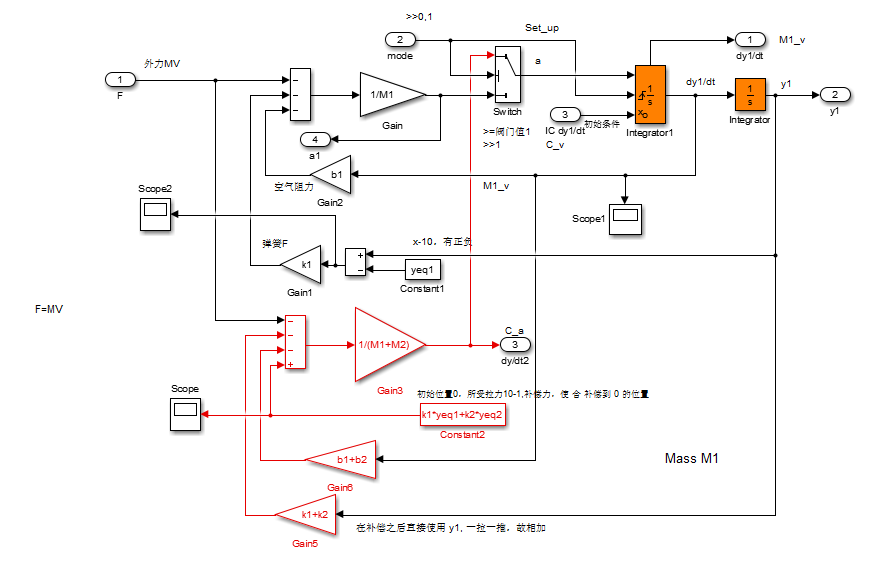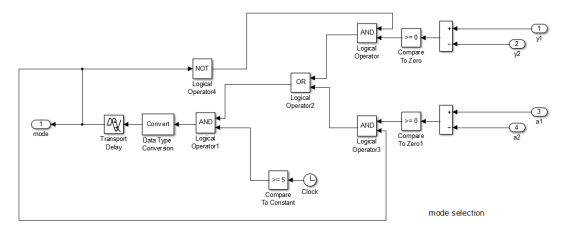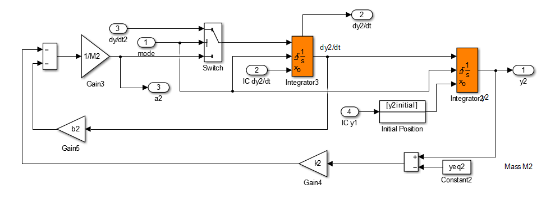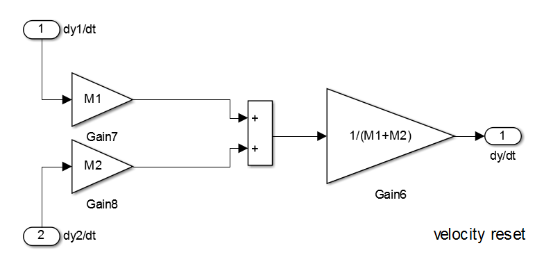The total simulation diagram上面的部分模拟两个物体的运动，并且这种移动会在在示波器中以信号的方式显示出来。并且当M1的位置和加速度均大于M2的位置和加速度时，model输出布尔量true或者false；下半部分包含以下要素:
1.多个MPC控制器,。这有四个输入：可测量输出(mo), 参考(ref)，可测量干扰(md)输入和独特的多个MPC控制器的功能块switch输入。
2.一脉冲发生器改变M1需要到达的位置 (控制器参考信号)。此脉冲发生器的输出是一个幅值为5的方波,频率是0.015每秒。

仿真过程展示：MPC1与MPC2协同工作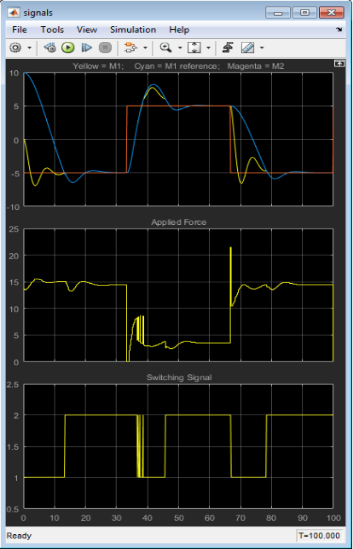大约在t = 13秒,M2与M1发生完全非弹性碰撞。M1与M2连在一起。第三幅图显示了开关信号的变化，在这个瞬间从1到2,所以MPC2开始起作用。。
在M1+M2超过了从其所期望的位置时候，M2和M1仍连在一起。控制器MPC2调整作用力F(中间的图片)使M1+M2快速返回到所需的位置。当r突变到5时，效果一样是非常不错的

%单个MPC1一直工作
%再进行一次无论在什么情况下只用MPC1系统的实验
disp('Now repeat simulation by using only MPC1 ...');
MPC2save=MPC2;     %先将MPC2保存起来
MPC2=MPC1;          %用MPC2来代替MPC21
sim('wc1',Tstop);  %动态系统仿真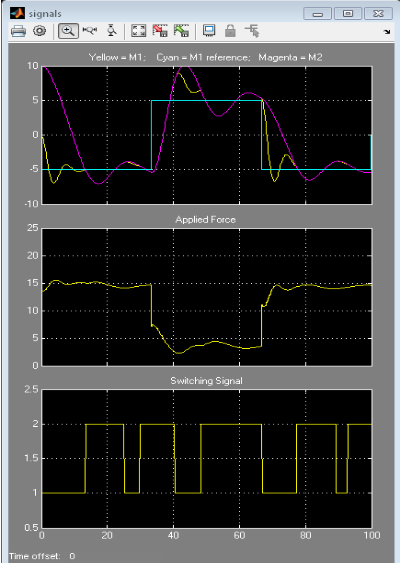%%如果一直是MPC1的系统，力F不够，当M1和M2链连接在一起的时候，移动缓慢,当下一个转变发生时还不能到达所需的位置

%单个MPC2一直工作
%再进行一次无论在什么情况下只用MPC2系统的实验
disp('Now repeat simulation by using only MPC2 ...');
MPC1=MPC2save;
MPC2=MPC1;
sim('WC1',Tstop);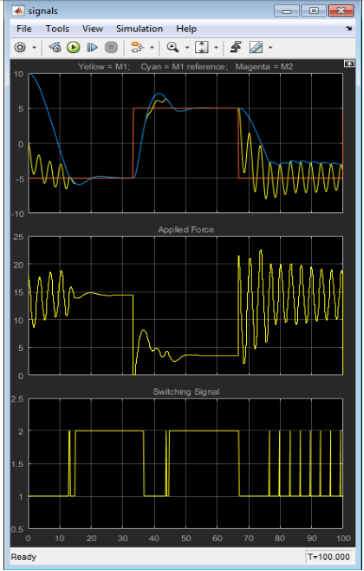%%当M1和M2分开的时候，MPC2也依然使用，过度使用F，过度补偿,导致振荡产生。当M2和M1连接在一起的时候,移动更平稳,和预期一样。最后过渡造成特别严重的振荡。 M1和M2频繁的碰撞,M1不能到达所需的位置

%%汇总
%% 多个MPC的的调度控制器
% clc
% clear all

%% 系统参数
clear all
clc
M1=1;       % M1的质量
M2=5;       % M2的质量
k1=1;       % 弹簧k1常数k
k2=0.1;     % 弹簧k2常数k
b1=0.3;     % 与空气的摩擦系数，与速度成正比
b2=0.8;     % 摩擦系数
yeq1=10;    % 刚性壁1的位置
yeq2=-10;   % 刚性壁2的位置

%% 状态空间模型
% 状态: M的速度和位置
% 被控变量:F
% 可测量的扰动:弹簧1的常熟；
% 测量输出: M1的位置

% 当M1和M2不接触时候的状态模型.
A1=[0 1;-k1/M1 -b1/M1];
B1=[0 0;-1/M1 k1*yeq1/M1];
C1=[1 0];
D1=[0 0];
sys1=ss(A1,B1,C1,D1);             %建立状态空间表达式
sys1=setmpcsignals(sys1,'MD',2)  ;%第二个输入是可测量扰动

% 当M1和M2接触时候的状态模型.
A2=[0 1;-(k1+k2)/(M1+M2) -(b1+b2)/(M1+M2)];
B2=[0 0;-1/(M1+M2) (k1*yeq1+k2*yeq2)/(M1+M2)];
C2=[1 0];
D2=[0 0];
sys2=ss(A2,B2,C2,D2);
sys2=setmpcsignals(sys2,'MD',2);

%% MPC仿真参数设置
Ts=0.2;     % 采样时间
p=20;       % 预测时域长度
m=1;        % 控制时域长度

%% 建立MPC模型
MPC1=mpc(sys1,Ts,p,m);                        % 定义M1和M2分离的MPC模型
MPC1.MV=struct('Min',0,'Max',Inf,'RateMin',-1e3,'RateMax',1e3);  % 设置限制，F的大小不能为0

MPC2=mpc(sys2,Ts,p,m);       %定义M1和M2连接时候的MPC模型
MPC2.MV=MPC1.MV;             %系统1和系统2的控制限制一样

%% 仿真参数设置
Tstop=100;      % 仿真时间
y1initial=0;    % 物体1的初始位置
y2initial=10;   % 物体2的初始位置
open_system('WC1');   % 调用WC1.MDL

%% 开始三次仿真
%双系统MPC1和MPC2仿真实验
disp('Start simulation by switching control between MPC1 and MPC2 ...');
set_param('wc1/signals','Open','On');%设置系统和模型的参数值
sim('WC1',Tstop);%动态系统仿真

%再进行一次无论在什么情况下只用MPC1系统的实验
disp('Now repeat simulation by using only MPC1 ...');
MPC2save=MPC2;  %先将MPC2保存起来
MPC2=MPC1;      %用MPC2来代替MPC21
sim('WC1',Tstop);

%再进行一次无论在什么情况下只用MPC2系统的实验
disp('Now repeat simulation by using only MPC2 ...');
MPC1=MPC2save;
MPC2=MPC1;
sim('WC1',Tstop);

%% 关闭仿真窗口
% bdclose('WC1')
% close(findobj('Tag','WC1_demo'))

%% reference
% A. Bemporad, S. Di Cairano, I. V. Kolmanovsky, and D. Hrovat, "Hybrid
%    modeling and control of a multibody magnetic actuator for automotive
%    applications," in Proc. 46th IEEE(R) Conf. on Decision and Control, New
%    Orleans, LA, 2007.
%http://cn.mathworks.com/help/mpc/gs/coordination-of-multiple-model-predictive-controllers.html#bs670e3-8
%李国勇，智能预测控制及其MATLAB实现.北京：电子工业出版社，2010.
posted @ 2018-05-11 22:05  |itsRae|  阅读(44447)  评论(7编辑  收藏  举报